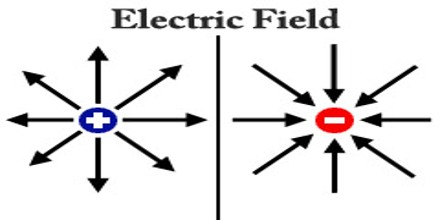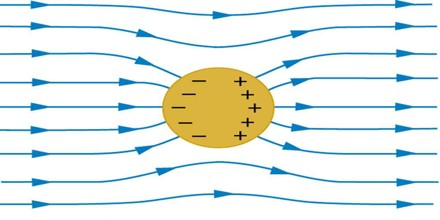Electric Field

Definition

Electric Field is a field of force that surrounding a charged particle within which another charged particle experiences a force Compare magnetic field. The strength of an electric field at a given point in space near an electrically charged object is proportional to the amount of charge on the object, and inversely proportional to the distance between the point and the object. The magnitude and direction of the electric field are expressed by the value of E, called electric field strength or electric field intensity or simply the electric field. Knowledge of the value of the electric field at a point, without any specific knowledge of what produced the field, is all that is needed to determine what will happen to electric charges close to that particular point.Electric field is also defined as the electric force per unit charge. The direction of the field is taken to be the direction of the force it would exert on a positive test charge. The electric field is radially outward from a positive charge and radially in toward a negative point charge. The electric field from any number of point charges can be obtained from a vector sum of the individual fields. A positive number is taken to be an outward field; the field of a negative charge is toward it.

The value of the electric field has dimensions of force per unit charge. In the metre-kilogram-second and SI systems, the appropriate units are newtons per coulomb, equivalent to volts per metre. In the centimetre-gram-second system, the electric field is expressed in units of dynes per electrostatic unit (esu), equivalent to statvolts per centimetre.Direction of Electric Field

Electrostatic force between two electrical charges is either repulsive or attractive depending upon the nature of charges; hence it is a vector quantity. So, electric field is a vector quantity, since electric field is the force per unit positive charge, so the direction of electrostatic field must follow the direction of electrostatic force. When, we place a unit positive charge in an electric field, it either comes closer or goes far, depending upon the nature of the charge, by which the field is created. The direction of the electric field is given by the direction of motion of the unit positive charge. Electric Field Intensity is always perpendicular to the surface.

Properties of Electric Field Lines

Electric Field Lines are two types –

• Uniform
• Non uniformBut for each type always follows some important mannerism as their characteristics or properties. They are-

• Electric field lines of force have a tendency to get separated from each other in the direction perpendicular to their lengths. They repel if they are of like charges.
• Electric lines of force start from the positively charged surface of a body and end negatively charged surface of a body.
• These lines of force are like elastic string, they comes to contract in length i.e. attract each other with respect to the opposite charges.
• Closeness of lines of forces symbolizes more strength of electric field and vice versa.
• Parallel lines indicate uniform field.
• Two lines of forces never intersect each other.
• Lines of force never pass through a conductor, i.e. field inside a conductor is always zero.
• The tangential direction at any point on the lines of forces indicates the direction of the force acting on the positive charge at that point.

Sources of Electric Field

Electric fields are caused by electric charges or varying magnetic fields. However, since the magnetic field is described as a function of electric field, the equations of both fields are coupled and together form Maxwell’s equations that describe both fields as a function of charges and currents. In the special case of a steady state (stationary charges and currents), the Maxwell-Faraday inductive effect disappears. The equations of electromagnetism are best described in a continuous description. However, charges are sometimes best described as discrete points; for example, some models may describe electrons as point sources where charge density is infinite on an infinitesimal section of space.Electric Dipole in Electric Field

When an electric dipole is placed inside a uniform electric field, the negative end of the dipole is attracted by positive end of the field and positive end of the dipole is attracted by negative end of the field. Due to these two forces, which are opposite in direction, there would be a torque acting on the dipole body. Let this torque is τ and θ is the angle between electric dipole and electric field. The amplitude of force acting on charge Q in the electric field E is given as EQ.

Electric Dipole is created by two opposite and equal charges, a certain distance apart. It is equal to the product of one charge and the distance between them. Due to this field the dipole will be oriented parallel to the electric field.